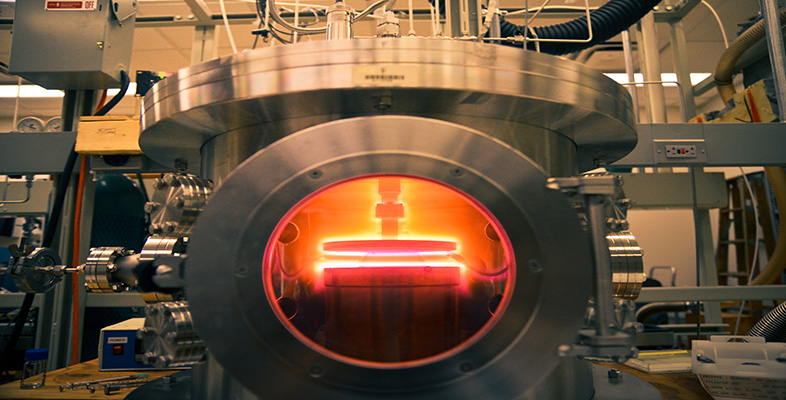Engineering: The challenge of temperature

Start this free course now. Just create an account and sign in. Enrol and complete the course for a free statement of participation or digital badge if available.

Free course

# 4 Accelerating temperature effects

## 4.1 Characteristics of processes activated by thermal energy

This is a long section and needs to be studied carefully. Keep your eye on the overall goal of seeking useful thermal effects on which to base devices.

This section continues the discussion of heat at an atomic level. You will need this background to appreciate the characteristics of processes activated by thermal energy – for example, the softening of glass in a gas flame, the diffusion of atoms through solids, the electrical conductivity of ceramics, and many chemical reactions. Such processes relate not only to the manipulation of materials, but also to the way in which the properties of materials vary with temperature. The exponential function y = exp(x) is an essential ingredient of the useful mathematical modelling that can be done in this case, so now is a good time to check you have the necessary background by reading Box 8 Exponentials and logarithms.

### Box 8 Exponentials and logarithms

Populations of rabbits are said to increase 'exponentially' if food is plentiful and predators are rare. Weed on a pond may appear to spread 'exponentially'. Money invested in a savings account appears to grow 'exponentially'. The mathematical function that can be fitted to all these scenarios is the exponential function y = exp(x); it is plotted in Figure 12(a). The function exp(x) is alternatively written as ex.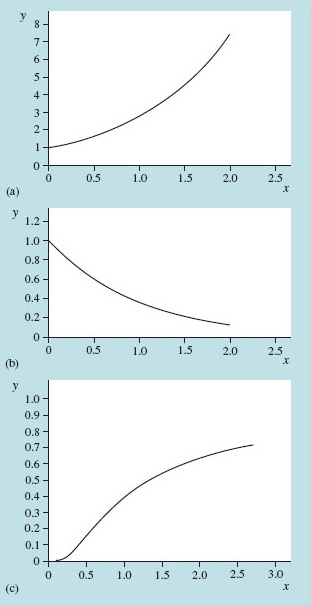Figure 12 Exponential functions: (a) y = exp(x), (b) y= exp(−x), (c)y = exp(−1/x)

On calculators the button marked EXP is to do with powers of ten and is not the exponential function. To get exp(x) the appropriate function key is usually the one labelled ex.

The usefulness of the exponential function goes beyond rabbits, pond weed and banking. It arises in science in many different situations. Often its occurrence can be traced to a key factor that changes in proportion to itself. Rabbits mature quickly and a large group reproduces in proportion to the number of rabbits. The potential of floating pond weed to capture energy from the sun and so grow in an almost empty pond is in proportion to the weed's existing surface area. The interest on a monthly savings account is earned in proportion to the amount in the account at the end of each month, so one month's interest adds to the initial sum as the basis for determining the next month's interest.

In these cases the model functions that relate the number of rabbits (N), the area of weed (α) and the balance of the savings account (B) to time are as follows: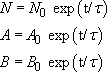in which the subscripts (zero) denote the values at time t = 0, and the symbol τ(Greek letter tau) is a 'characteristic time'. The exponential function equals one (1.0) when the argument (here t/τ) is zero. At the time when t = τ, the argument is 1.0 and the function equals the mathematical constant 'e', which has a value of 2.718 … .

A similar exponential function describes the decay of a population that randomly dies out. For example, consider a large number of radioactive atoms that spontaneously undergo a decay, emitting one of the characteristic types of radiation (alpha, beta and gamma). Once any radioactive atom has 'emitted' its radioactivity it is, so to speak, spent. In any brief interval of time, the number of atoms that 'decay' depends simply on the number that remain. From this follows an exponential function that describes a slowing decline in activity of the original group with time (see Figure 12b):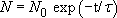Notice the negative argument (−t/τ) of the exponential function in this case. The decay of electrical charge in a leaking capacitor follows a similar trend, as does the temperature of a cup of tea relative to that of its surroundings. In each case the rate of loss of charge or heat is proportional to the amount that remains.

To 'undo' an exponential function we take natural logarithms (ln). So, to recover x from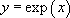you must take the natural logarithm of both sides of the equation: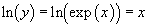That amounts to a definition of the natural logarithm function ln(y).

(On calculators natural logarithms are usually performed by means of the function key marked either 'In' or 'log e'.)

Another occasion when the exponential function arises is in connection with the statistics of very large populations of identical particles. That means we may expect to see it when discussing several million million million atoms. In this context the argument of the exponential is usually some particular, fixed energy E divided by a thermal energy kT, and it is negative: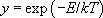This form of the exponential is very important in the context of thermally accelerating effects. Figure 12(c) shows how the exponential function looks when the variable is 'upside down inside a negative exponential', as T is in this last equation. In this case the curve rises increasingly rapidly at first, but later flattens out.